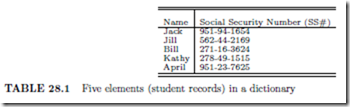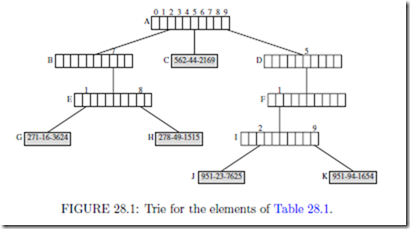# Tries:What Is a Trie?

What Is a Trie?

A trie (pronounced “try” and derived from the word retrieval) is a data structure that uses the digits in the keys to organize and search the dictionary. Although, in practice, we can use any radix to decompose the keys into digits, in our examples, we shall choose our radixes so that the digits are natural entities such as decimal digits (0, 1, 2, 3, 4, 5, 6, 7, 8, 9) and letters of the English alphabet (a z, A Z).

Suppose that the elements in our dictionary are student records that contain ﬁelds such as student name, major, date of birth, and social security number (SS#). The key ﬁeld is the social security number, which is a nine digit decimal number. To keep the example manageable, assume that the dictionary has only ﬁve elements. Table 28.1 shows the name and SS# ﬁelds for each of the ﬁve elements in our dictionary.

To obtain a trie representation for these ﬁve elements, we ﬁrst select a radix that will be used to decompose each key into digits. If we use the radix 10, the decomposed digits arejust the decimal digits shown in Table 28.1. We shall examine the digits of the key ﬁeld (i.e., SS#) from left to right. Using the ﬁrst digit of the SS#, we partition the elements into three groups–elements whose SS# begins with 2 (i.e., Bill and Kathy), those that begin with 5 (i.e., Jill), and those that begin with 9 (i.e., April and Jack). Groups with more than one element are partitioned using the next digit in the key. This partitioning process is continued until every group has exactly one element in it.

The partitioning process described above naturally results in a tree structure that has 10-way branching as is shown in Figure 28.1. The tree employs two types of nodes–branch nodes and element nodes. Each branch node has 10 children (or pointer/reference) ﬁelds.

These ﬁelds, child[0 : 9], have been labeled 0, 1, ··· , 9 for the root node of Figure 28.1.

root.child[i] points to the root of a subtrie that contains all elements whose ﬁrst digit is i.

In Figure 28.1, nodes A, B, D, E, F, and I are branch nodes. The remaining nodes, nodes C, G, H, J, and K are element nodes. Each element node contains exactly one element of the dictionary. In Figure 28.1, only the key ﬁeld of each element is shown in the element nodes